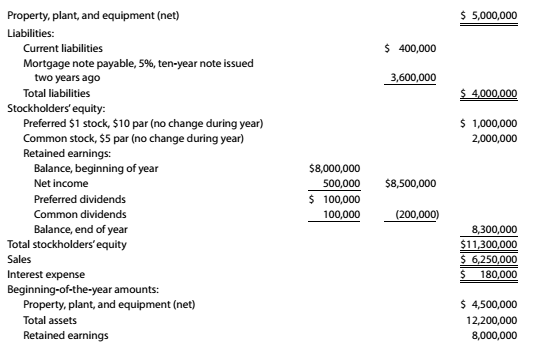Chapter 9, Problem 9.19E

Chapter
Section
Textbook Problem

Seven metrics The following data were taken from the financial statements of Woodwork Enterprises Inc. for the current fiscal year. Assuming that there are no intangible assets, determine the following: (a) debt ratio, (b) ratio of fixed assets to long-term liabilities, (c) ratio of liabilities to stockholders' equity, (d) asset turnover, (e) return on total assets, (f) return on stockholders' equity, and (g) return on common stockholders' equity. Round to two decimal places.To determine

(a)

Debit ratio:

It measures the extents of companies leverage.It can be interpreted as proportion of company's assets financed by debts. It can be calculated by:

Debit ratio= total debttotal assets

To calculate:

The debit ratio.

Explanation

Total Liabilities = $4,000,000 Total Stockholders' Equity =$11,300,000

Total Assets = Total Liabilities + Total Stockholders' Equity

Total Assets = $4,000,000 +$11,300,000 = \$15,300,000

Debt Ratio = &

To determine

(b)

Concept Introduction:

Fixed assets to long term liabilities: it is a way to measure solvency bigger number is considered as better low number indicates that fixed assets may not be sufficient to cover long term debts

Fixed assets to long term liabilities= fixed assetslong term liabilities

To calculate:

Ratio of fixed assets to long term liabilities.

To determine

(c)

Concept Introduction:

Ratio of liabilities to shareholders' equity or debt to equity ratio:

It is used to evaluate company's financial leverage. It reflects the ability of shareholders equity to cover all outstanding debts. It can be calculated as follows:

Debt to equity ratio= total debttotal shareholders equity

Ratio of liabilities to shareholders' equity.

To determine

(d)

Concept Introduction:

Assets turnover:

It indicates the efficiency with which the company use its assets to generate revenue.

Higher asset turnover indicate more efficient utilisation of assets.

Assets turnover=Sales revenue  Average total Assets

To calculate:

Assets turnover ratio.

To determine

(e)

Concept Introduction:

Profitability analysis:

It is used to evaluate the ability of a company to earn income in relation to assets, operating cost and shareholders' equity during a specific period.

Return on total assets shows the total return on investment made by the firm.

To calculate:

Return on total assets.

To determine

(f)

Concept Introduction:

Profitability analysis:

It is used to evaluate the ability of a company to earn income in relation to assets, operating cost and shareholders' equity during a specific period.

Return on shareholders' equity shows total return on investment by shareholders.

Return on shareholders' equity

To determine

(g)

Concept Introduction:

Profitability analysis:

It is used to evaluate the ability of a company to generate income relative to revenue, assets, operating cost and shareholders' equity during a specific period.

Return on common shareholders' equity shows total return on investment by common shareholders.

To calculate:

Return on common shareholders' equity.

Still sussing out bartleby?

Check out a sample textbook solution.

See a sample solution

The Solution to Your Study Problems

Bartleby provides explanations to thousands of textbook problems written by our experts, many with advanced degrees!

Get Started

Define small data analytics.

Accounting Information Systems

What is product diversity?

Managerial Accounting: The Cornerstone of Business Decision-Making

CURRENT RATIO The Petry Company has 1312,500 in current assets and 525,000 in current liabilities. Its initial ...

Fundamentals of Financial Management, Concise Edition (with Thomson ONE - Business School Edition, 1 term (6 months) Printed Access Card) (MindTap Course List)

2. What does a share of stock represent?

Cornerstones of Financial Accounting